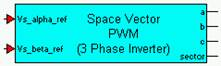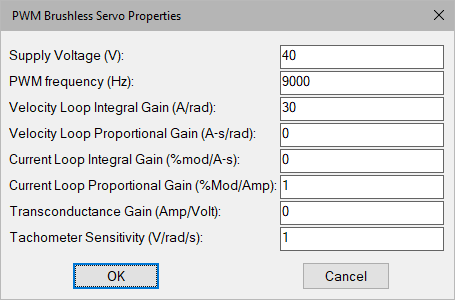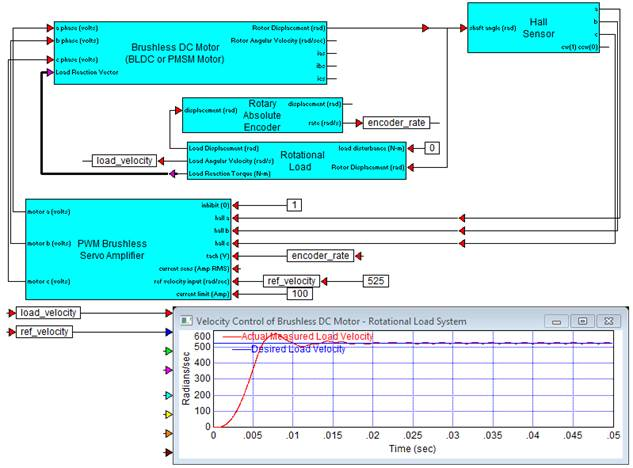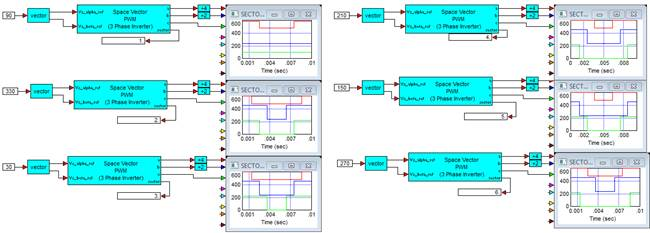##### PWM (Space Vector)Category: eMotors > Other Tools > PWM

The PWM (Space Vector) block <tbs. will the parentheses be removed> produces PWM pulses from orthogonal 2-phase input signals using space vector modulation techniques

vSampling Rate: Defines the processor rate for PWM calculations in hertz. Note that this frequency is typically chosen to be large (8000 Hz or greater). The simulation rate should be at least 10 times the PWM rate to assure proper operation of the simulation.

DC Bus Voltage: Defines the output voltage level of the 3-phase PWM signals in volts.

#### Example

Diagram name: PWM Space Vector

Location: Examples > eMotors > Transforms

Modulation patterns in SVPWM occur based on the location of the timing vector in 6 possible sectors in one complete revolution. The diagram is constructed and duplicated 6 times, changing the input angle in 60 degree increments for each diagram copy that places the timing vector mid-sector for each case. Output phases are then connected to plot blocks to display the patterns for each sector, as shown in the following diagram.